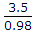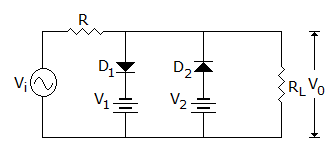# Electronics and Communication Engineering - Analog Electronics

1.

A CB amplifier has a = 0.98 and RL = 600 Ω. If IE = 3.5 mA, the current gain is

 A. 1 B. 0.98 C. 0.98 x 3.5 D.Explanation:

Current gain of CB amplifier is a.

2.

funity of an op-amp is about 1 kHz.

 A. True B. False

Explanation:

3.

Assertion (A): In an RC coupled amplifier the junction capacitances can be neglected at low frequencies but not at high frequencies

Reason (R): Capacitive reactance is inversely proportional to frequency.

 A. Both A and R are correct and R is correct explanation for A B. Both A and R are correct but R is not correct explanation for A C. A is correct R is wrong D. A is wrong R is correct

Explanation:

At high frequencies XC is very small.

4.

In figure, D1 turns on whenA. vi is more than V1 B. vi is less than V1 C. vi is between V1 and V2 D. none of the above

Explanation:

D1 is forward biased when vi > V1.

5.

In a full wave rectifier circuit using centre tapped transformer, the peak voltage across half of the secondary winding is 30 V. Then PIV is

 A. 30 V B. 60 V C. 15 V D. 10 V